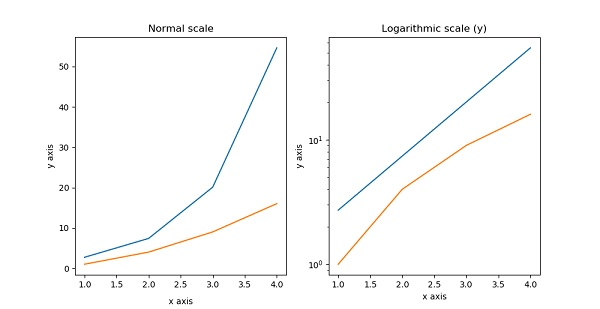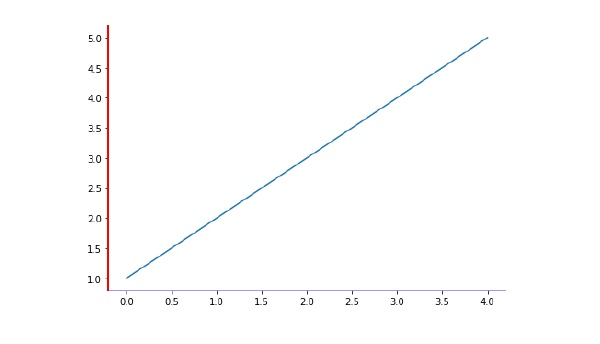# Matplotlib - Formatting Axes

Sometimes, one or a few points are much larger than the bulk of data. In such a case, the scale of an axis needs to be set as logarithmic rather than the normal scale. This is the Logarithmic scale. In Matplotlib, it is possible by setting xscale or vscale property of axes object to ‘log’.

It is also required sometimes to show some additional distance between axis numbers and axis label. The labelpad property of either axis (x or y or both) can be set to the desired value.

Both the above features are demonstrated with the help of the following example. The subplot on the right has a logarithmic scale and one on left has its x axis having label at more distance.

```import matplotlib.pyplot as plt
import numpy as np
fig, axes = plt.subplots(1, 2, figsize=(10,4))
x = np.arange(1,5)
axes.plot( x, np.exp(x))
axes.plot(x,x**2)
axes.set_title("Normal scale")
axes.plot (x, np.exp(x))
axes.plot(x, x**2)
axes.set_yscale("log")
axes.set_title("Logarithmic scale (y)")
axes.set_xlabel("x axis")
axes.set_ylabel("y axis")
axes.set_xlabel("x axis")
axes.set_ylabel("y axis")
plt.show()
```Axis spines are the lines connecting axis tick marks demarcating boundaries of plot area. The axes object has spines located at top, bottom, left and right.

Each spine can be formatted by specifying color and width. Any edge can be made invisible if its color is set to none.

```import matplotlib.pyplot as plt
fig = plt.figure()
ax.spines['bottom'].set_color('blue')
ax.spines['left'].set_color('red')
ax.spines['left'].set_linewidth(2)
ax.spines['right'].set_color(None)
ax.spines['top'].set_color(None)
ax.plot([1,2,3,4,5])
plt.show()
```